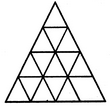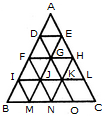# Non Verbal Reasoning - Analytical Reasoning - Discussion

Discussion Forum : Analytical Reasoning - Section 1 (Q.No. 14)
14.

Find the minimum number of straight lines required to make the given figure.9
11
15
16
Explanation:

The figure may be labelled as shown.The horizontal lines are DE, FH, IL and BC i.e. 4 in number.

The slanting lines are AC, DO, FN, IM, AB, EM and HN i.e. 7 in number.

Thus, there are 4 + 7 = 11 straight lines in the figure.

Discussion:
5 comments Page 1 of 1.

Surya prakash said:   4 years ago
Triangle is 16.

Umar said:   5 years ago
But the real triangle exist is 15.

Bidur said:   7 years ago
Anyone can say, how many numbers of a triangle are there?